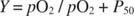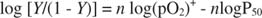## Hemoglobin and Myoglobin

Hemoglobin and myoglobin are only slightly related in primary sequence. Although most amino acids are different between the two sequences, the amino acid changes between the two proteins are generally conservative. More strikingly, the secondary structures of myoglobin and the subunits of hemoglobin are virtually identical, as shown in Figure . Both proteins are largely alpha‐helical, and the helices fit together in a similar way. One O 2 molecule is bound to each protein molecule by a coordinate covalent bond to an iron atom (Fe(II)) in the heme group . Heme is a square planar molecule containing four pyrrole groups, whose nitrogens form coordinate covalent bonds with four of the iron's six available positions. One position is used to form a coordinate covalent bond with the side chain of a single histidine amino acid of the protein, called the proximal histidine. The sixth and last orbital is used for oxygen. It is empty in the nonoxygenated forms of hemoglobin and myoglobin.Figure 1

When molecular oxygen encounters an isolated heme molecule, it rapidly converts the Fe(II) to Fe(III). The oxidized heme binds oxygen very poorly. Obviously, if this happened to the Fe(II) groups of hemoglobin and myoglobin, the proteins would be less useful as oxygen carriers. Oxidation of the heme iron is prevented by the presence of the distal histidine side chain, which prevents the O 2 from forming a linear Fe–O–O bond. The bond between Fe and O 2 is bent, meaning that this bond is not as strong as it might be. Weaker oxygen binding means easier oxygen release. This is an important principle in understanding not only heme chemistry but also the regulation of hemoglobin's affinity for oxygen.

The differences between hemoglobin and myoglobin are most important at the level of quaternary structure. Hemoglobin is a tetramer composed of two each of two types of closely related subunits, alpha and beta. Myoglobin is a monomer (so it doesn't have a quaternary structure at all). Myoglobin binds oxygen more tightly than does hemoglobin. This difference in binding energy reflects the movement of oxygen from the bloodstream to the cells, from hemoglobin to myoglobin.

## Myoglobin binds oxygen The binding of O 2 to myoglobin is a simple equilibrium reaction:Each myoglobin molecule is capable of binding one oxygen, becausemyoglobin contains one heme per molecule. Even though the reaction of myoglobin and oxygen takes place in solution, it is convenient to measure the concentration of oxygen in terms of its partial pressure, the amount of gas in the atmosphere that is in equilibrium with the oxygen in solution.

The titration curve of myoglobin with oxygen is a hyperbola, as shown in Figure of the form:where Y is the fraction of oxygenated myoglobin, pO 2 is the partial pressure of O 2, expressed in torr (mm Hg; 760 torr = 1 atmosphere) and P 0 is the partial pressure of O 2 required to bind 50% of the myoglobin molecules. The derivation of this equation from the equilibrium constant for binding is not reproduced here but may be found in many standard chemistry and biochemistry textbooks. In the above equation, if Y is set at 0.5, P 50 = pO 2.Figure 2

Under standard physiological conditions, the P 50 for O 2 binding to myoglobin is approximately 1 torr. Because the atmosphere is approximately 21% oxygen, at 1 atmosphere of pressure, pO 2 = 0.21 × 760 = 159 torr myoglobin would be almost totally saturated:Because pO 2 in blood is lower than atmospheric pO 2, Y varies from 0.91 (venous blood) to 0.97 (arterial blood).

## Binding oxygen to hemoglobin

Because hemoglobin has four subunits, its binding of oxygen can reflect multiple equilibria:The equilibrium constants for these four O 2 binding events are dependent on each other and on the solution conditions. The influence of one oxygen's binding on the binding of another oxygen is called a homotropic effect. Overall, this cooperative equilibrium binding makes the binding curve sigmoidal rather than hyperbolic, as Figure shows. The P 50 of hemoglobin in red blood cells is about 26 torr under normal physiological conditions. In the alveoli of the lungs, pO 2 is about 100 torr, and close to 20 torr in the tissues. So you may expect hemoglobin to be about 80% loaded in the lungs and a little over 40% loaded with oxygen in the tissue capillaries. In fact, hemoglobin can be more O 2‐saturated in the lungs and less saturated in the capillaries.Figure 3

Cooperativity is a complex subject; one model is the interconversion of the hemoglobin between two states—the T (tense) and the R (relaxed) conformations—of the molecule. The R state has higher affinity for oxygen. Under conditions where pO 2 is high (such as in the lungs), the R state is favored; in conditions where pO 2 is low (as in exercising muscle), the T state is favored.

Quantitatively, the binding curve of a complex protein like hemoglobin is described by the approximation:where n is the number of interacting subunits. The equation can be manipulated into logarithmic form:A graph of this equation yields a Hill plot (named after the British physiologist who first described it), as shown in Figure . Hill plots of log [ Y/(1 ‐ Y)] versus log(pO 2) are linear, over at least part of the range, with slope n (sometimes called a Hill coefficient), which is the minimal number of interacting subunits. For myoglobin, which only has one subunit, the slope must be 1; for hemoglobin, the Hill coefficient is 2.8, indicating a minimum of three interacting subunits, although there are four subunits in a molecule of hemoglobin.

Even if no other factors operated, because the P 50 of hemoglobin is greater than the P 50 for myoglobin, hemoglobin in the blood gives up O 2 to myoglobin in the tissues. This follows from the principle discussed above, that a greater P 50 means lower affinity, and weaker binding means greater release.